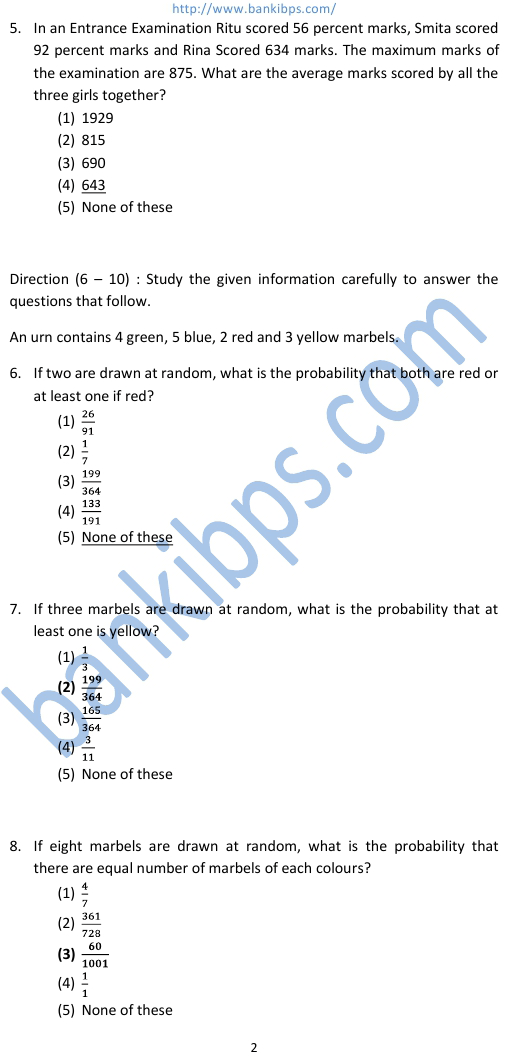1. Seema bought 20 pens, 8 packets of wax colours, 6 calculators and 7 pencils boxes. The price of 1 pen is Rs 7, one packet of wax colour is Rs 22, one calculator is Rs 175 and one pencil box is Rs 14 more than the combined price of one pen and one packet of wax colours. How much amount did Seema pay to the shopkeeper? (1) Rs 1491 (2) Rs 1725 (3) Rs 1667 (4) Rs 1527 (5) None of these ibps po previous question papers with answers download 2. The average marks in English subject of a class of 24 students is 56. If the marks of three students were misread as 44, 45 and 61 of the actual marks 48, 59 and 67 respectively, then what would be the correct average? (1) 56.5 (2) 59 (3) 57.5 (4) 58 (5) None of these 3. In a test, a candidate secured 468 marks out of maximum marks ‘A’. if the maximum marks ‘A’ were converted to 700 marks, he would have secured 336 marks. What were the maximum marks of the test? (1) 775 (2) 875 (3) 975 (4) 1075 (5) None of these 4. Six-eleventh of a number is equal to twenty two percent of second number. Second number is equal to the one-forth of third number. The value of the third number is 2400. What is the 45% of the number? (1) 109.8 (2) 111.7 (3) 117.6 (4) 123.4 (5) None of these